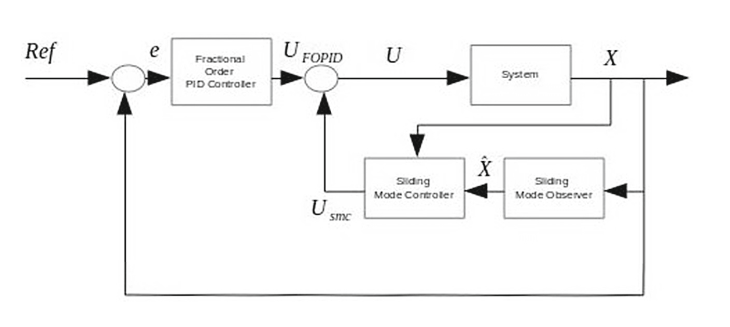Conference Paper

# Fractional Order Sliding Mode PID Controller/Observer for Continuous Nonlinear Switched Systems with PSO Parameter Tuning

By
Azar A.T.
Serrano F.E.

In this article a fractional order sliding mode PID controller and observer for the stabilization of continuous nonlinear switched systems is proposed. The design of the controller and observer is done following the separation principle, this means that the observer and controller are designed in a separate fashion, so a hybrid controller is implemented by designing the sliding mode controller part using an integral sliding mode surface along with a PIλDμ controller part which is the fractional order PID controller that is implemented to stabilizes the system. For the observer part, an integral sliding manifold is implemented, so the error between the measured and estimated variable is reduced to zero when time approaches to infinity. The fractional order PID part is tuned by particle swarm optimization algorithm to derive the λ and μ parameters along with the gain matrices. Finally, the stability of the closed loop system is assured in synchronous switching, this means that the mode in the controller and the system is the same at every switching time, the average dwell time is implemented for this purpose. © 2018, Springer International Publishing AG.